Home | | Physics 11th std | Torque and Angular Momentum

# Torque and Angular Momentum

When a net force acts on a body, it produces linear motion in the direction of the applied force.

TORQUE AND ANGULAR MOMENTUM

When a net force acts on a body, it produces linear motion in the direction of the applied force. If the body is fixed to a point or an axis, such a force rotates the body depending on the point of application of the force on the body. This ability of the force to produce rotational motion in a body is called torque or moment of force. Examples for such motion are plenty in day to day life. To mention a few; the opening and closing of a door about the hinges and turning of a nut using a wrench.

The extent of the rotation depends on the magnitude of the force, its direction and the distance between the fixed point and the point of application. When torque produces rotational motion in a body, its angular momentum changes with respect to time. In this Section we will learn about the torque and its effect on rigid bodies.

## Definition of Torque

Torque is defined as the moment of the external applied force about a point or axis of rotation. The expression for torque is,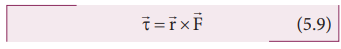where,is the position vector of the point where the forceis acting on the body as shown in Figure 5.4.Here, the product ofandis called the vector product or cross product. The vector product of two vectors results in another vector that is perpendicular to both the vectors (refer Section 2.5.2). Hence, torque) is a vector quantity.

Torque has a magnitude ( rFsinθ ) and direction perpendicular toand. Its unit is N m.Here, θ is the angle betweenand, and nˆ is the unit vector in the direction of. Torque () is sometimes called as a pseudo vector as it needs the other two vectorsandfor its existence.

The direction of torque is found using right hand rule. This rule says that if fingers of right hand are kept along the position vector with palm facing the direction of the force and when the fingers are curled the thumb points to the direction of the torque. This is shown in Figure 5.5.

The direction of torque helps us to find the type of rotation caused by the torque. For example, if the direction of torque is out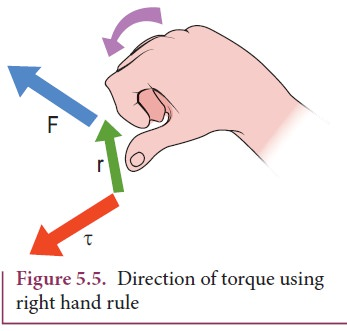of the paper, then the rotation produced by the torque is anticlockwise. On the other hand, if the direction of the torque is into the paper, then the rotation is clockwise as shown in Figure 5.6.In many cases, the direction and magnitude of the torque are found separately. For direction, we use the vector rule or right hand rule. For magnitude, we use scalar form as,The expression for the magnitude of torque can be written in two different ways by associating sin θ either with r or F in the following manner.Here, (F sinθ) is the component ofperpendicular to. Similarly, ( r sinθ) is the component ofperpendicular to. The two cases are shown in Figure 5.7.In the earlier sections, we have dealt with the torque about a point. In this section we will deal with the torque about an axis. Let us consider a rigid body capable of rotating about an axis AB as shown in Figure 5.8. Let the force F act at a point P on the rigid body. The force F may not be on the plane ABP. We can take the origin O at any random point on the axis AB.Th e torque of the forceabout O is,=x. Th e component of the torque along  the axis is the torque ofabout the axis. To find it, we should first find the vector= |x| and then find the angle φ betweenand AB. (Remember here,is not on the plane ABP). Th e torque about AB is the parallel component of the torque along AB, which is |x| cos φ. And the torque perpendicular to the axis AB is |x| sin φ|.

The torque about the axis will rotate the object about it and the torque perpendicular to the axis will turn the axis of rotation. When both exist simultaneously on a rigid body, the body will have a precession. One can witness the precessional motion in a spinning top when it is about to come to rest as shown in Figure 5.9.Study of precession is beyond the scope of the higher secondary physics course. Hence, it is assumed that there are constraints to cancel the effect of the perpendicular components of the torques, so that the fixed position of the axis is maintained. Therefore, perpendicular components of the torque need not be taken into account.

Hereafter, for the calculation of torques on rigid bodies we will:

a.        Consider only those forces that lie on planes perpendicular to the axis (and do not intersect the axis).

b.        Consider position vectors which are perpendicular to the axis.

The torque of a force about an axis is independent of the choice of the origin as long as it is chosen on that axis itself. This can be shown as below.

Let O be the origin on the axis AB, which is the rotational axis of a rigid body. F is the force acting at the point P. Now, choose another point O’ anywhere on the axis as shown in Figure 5.10.The torque of F about O’ is,## Torque and Angular Acceleration

Let us consider a rigid body rotating about a fixed axis. A point mass m in the body will execute a circular motion about a fixed axis as shown in Figure 5.11. A tangential forceacting on the point mass produces the necessary torque for this rotation. This forceis perpendicular to the position vectorof the point mass.The torque produced by the force on the point mass m about the axis can be written as,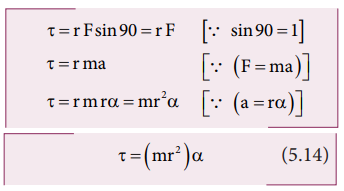Hence, the torque of the force acting on the point mass produces an angular acceleration ( α) in the point mass about the axis of rotation.

In vector notation,The directions of τ and α are along the axis of rotation. If the direction of τ is in the direction of α, it produces angular acceleration. On the other hand if, τ is opposite to α, angular deceleration or retardation is produced on the point mass.

The term mr2 in equations 5.14 and 5.15 is called moment of inertia (I) of the point mass. A rigid body is made up of many such point masses. Hence, the moment of inertia of a rigid body is the sum of moments of inertia of all such individual point masses that constitute the body ( I = mi ri2 ). Hence, torque for the rigid body can be written as,We will learn more about the moment of inertia and its significance for bodies with different shapes in section 5.4.

## Angular Momentum

The  angular  momentum  in  rotational motion is equivalent to linear momentum in  translational  motion.  The  angular momentum of a point mass is defined as the moment of its linear momentum. In other words, the angular momentum L of a point mass having a linear momentum p at a position r with respect to a point or axis is mathematically written as,The magnitude of angular momentum could be written as,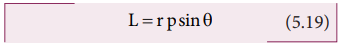where, θ is the angle betweenandis perpendicular to the plane containingand. As we have written in the case of torque, here also we can associate sin θ with eitheror.where, p is the component of linear momentum p perpendicular to r, and r is the component of position r perpendicular to p.

The angular momentum is zero ( L = 0 ), if the linear momentum is zero (p = 0) or if the particle is at the origin (= 0) or ifandare parallel or antiparallel to each other (0 0 or 1800).

There is a misconception that the angular momentum is a quantity that is associated only with rotational motion. It is not true. The angular momentum is also associated with bodies in the linear motion. Let us understand the same with the following example.

## Angular Momentum and Angular Velocity

Let us consider a rigid body rotating about a fixed axis. A point mass m in the body will execute a circular motion about the fixed axis as shown in Figure 5.12.The point mass m is at a distance r from the axis of rotation. Its linear momentum at any instant is tangential to the circular path. Then the angular momentumis perpendicular toand. Hence, it is directed along the axis of rotation. The angle θ betweenandin this case is 90o. The magnitude of the angular momentum L could be written as,

L = rmv sin90o = rmvwhere, v is the linear velocity. The relation between linear velocity v and angular velocity ω in a circular motion is, v = r ω. Hence,The directions of L and ω are along the axis of rotation. The above expression can be written in the vector notation as,As discussed earlier, the term mr2 in equations 5.22 and 5.23 is called moment of inertia (I) of the point mass. A rigid body is made up of many such point masses. Hence, the moment of inertia of a rigid body is the sum of moments of inertia of all such individual point masses that constitute the body ( I = mi ri 2 ). Hence, the angular momentum of the rigid body can be written as,The study about moment of inertia (I) is reserved for Section 5.4.

## Torque and Angular Momentum

We have the expression for magnitude of angular momentum of a rigid body as, L = I ω . The expression for magnitude of torque on a rigid body is, τ = I α

We can further write the expression for torque as,Where, ω is angular velocity and a is angular acceleration. We can also write equation 5.26 as,The above expression says that an external torque on a rigid body fixed to an axis produces rate of change of angular momentum in the body about that axis.

This is the Newton’s second law in rotational motion as it is in the form of F = dp/dt which holds good for translational motion.

### Conservation of angular momentum:

From the above expression we could conclude that in the absence of external torque, the angular momentum of the rigid body or system of particles is conserved.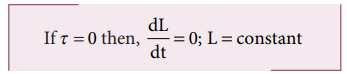The above expression is known as law of conservation of angular momentum. We will learn about this law further in section 5.5.

Solved Example Problems for Torque

### Example 5.7

If the force applied is perpendicular to the handle of the spanner as shown in the diagram, find the (i) torque exerted by the force about the center of the nut, (ii) direction of torque and (iii) type of rotation caused by the torque about the nut.### Solution

Arm length of the spanner, r = 15 cm = = 15×10−2m

Force, F = 2.5 N

Angle between r and F, θ = 90o(i) Torque, τ θ = rF sin(ii) As  per  the  right  hand  rule,  the direction of torque is out of the page.

(iii) The type of rotation caused by the torque is anticlockwise.

### Example 5.8### Example 5.9

A crane has an arm length of 20 m inclined at 30o with the vertical. It carries a container of mass of 2 ton suspended from the top end of the arm. Find the torque produced by the gravitational force on the container about the point where the arm is fixed to the crane. [Given: 1 ton = 1000 kg; neglect the weight of the arm. g = 10 ms-2]### Solution

The force F at the point of suspension is due to the weight of the hanging mass.We can solve this problem by three different methods.

Method – I

The angle (θ) between the arm length (r) and the force (F) is, θ = 150o

The torque (τ) about the fixed point of the arm is,Method – II

Let us take the force and perpendicular distance from the point where the arm is fixed to the crane.Method – III

Let us take the distance from the fixed point and perpendicular force.All the three methods, give the same answer

Solved Example Problems for Torque about an Axis

### Example 5.10

Three mutually perpendicular beams AB, OC, GH are fixed to form a structure which is fixed to the ground firmly as shown in the Figure. One string is tied to the point C and its free end D is pulled with a force F. Find the magnitude and direction of the torque produced by the force,

i. about the points D, C, O and B

ii. about the axes CD, OC, AB and GH.### SolutionThe torque of a force about an axis is independent of the choice of the origin as long as it is chosen on that axis itself. Th is can be shown as below.

Let O be the origin on the axis AB, which is the rotational axis of a rigid body. F is the force acting at the point P. Now, choose another point O’ anywhere on the axis as shown in Figure 5.10.Solved Example Problems for Angular Momentum

### Example 5.11

A particle of mass (m) is moving with constant velocity (v). Show that its angular momentum about any point remains constant throughout the motion.

### SolutionThe term ( OQsinθ ) is the perpendicular distance (d) between the origin and line along which the mass is moving. Hence, the angular momentum of the particle about the origin is,The above expression for angular momentum L, does not have the angle θ. As the momentum (p = mv) and the perpendicular distance (d) are constants, the angular momentum of the particle is also constant. Hence, the angular momentum is associated with bodies with linear motion also. If the straight path of the particle passes through the origin, then the angular momentum is zero, which is also a constant.

Tags : Definition, Formula, Equation, Comparision, Solved Example Problems , 11th Physics : Motion of System of Particles and Rigid Bodies
Study Material, Lecturing Notes, Assignment, Reference, Wiki description explanation, brief detail
11th Physics : Motion of System of Particles and Rigid Bodies : Torque and Angular Momentum | Definition, Formula, Equation, Comparision, Solved Example Problems

Related Topics